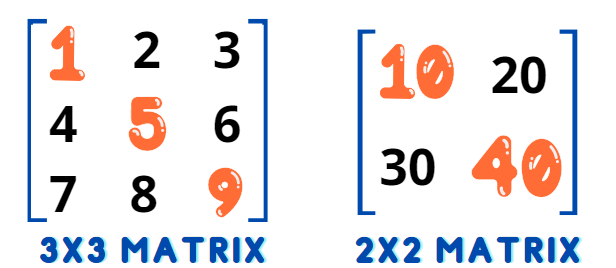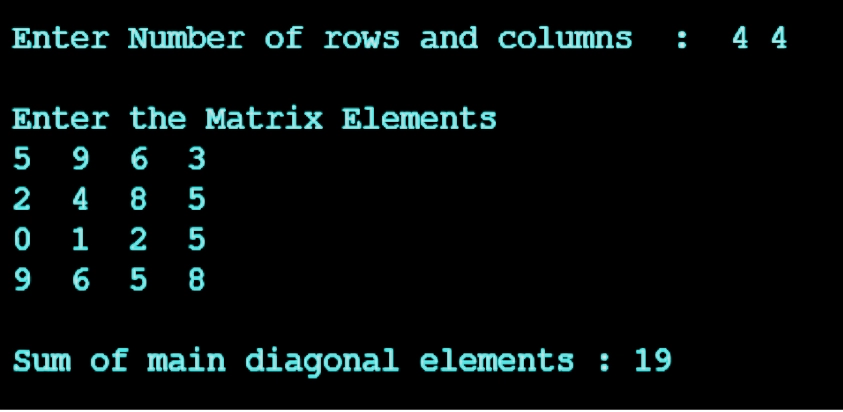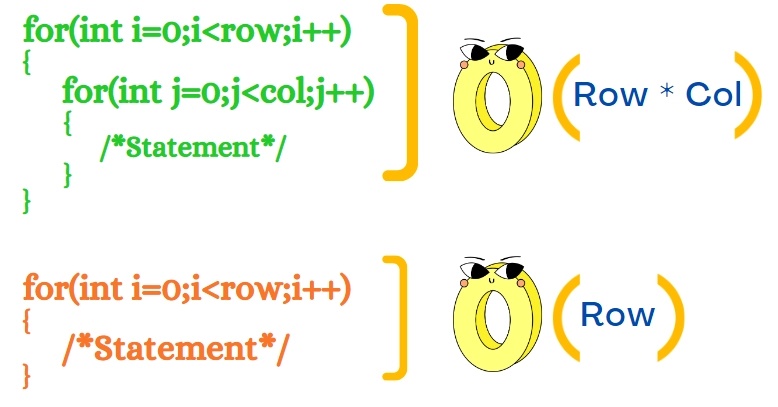New update is available. Click here to update.

# C Program to Find the Sum of Main Diagonal Elements of a Matrix

## Introduction

In this article, we will discuss a C program to find the sum of main diagonal elements of a matrix, the algorithm, the approach used to solve this problem, and its time and space complexities.

Before discussing the C program to find the sum of main diagonal elements of a matrix, let’s first discuss what is meant by a matrix and its main diagonal.

Matrix: A matrix is an array or table of numbers arranged in rows and columns, usually denoted by a capital letter.

Main Diagonal of Matrix: The diagonal of a matrix represents the elements present diagonally in the matrix. The elements from the top left corner to the bottom right corner represent the main diagonal.

For Example:We have taken a 2x2 matrix and a 3x3 matrix, and the colored elements represent the main diagonal elements of the matrix.

In this 3x3 matrix, the sum of main diagonal elements is = 1 + 5 + 9 = 15

Similarly, the sum of diagonal elements in the 2x2 matrix is = 10 + 40 = 50

## Problem StatementWe need to write a  C program to find the sum of main diagonal elements of a matrix by reading the size and elements of the matrix from the user, adding the elements of the main diagonal, and finally returning that sum. Also, check if the matrix is a square matrix or not. If it is not a square matrix, give a warning message and again execute from starting. Also, you can implement a code with the help of online editor for good practice.

## Logic

The logic is almost the same as the sum of all the elements of a matrix, the only difference is that here we add only those elements of the matrix for which column number and row number are same, like the element present at (1,1) i.e., 1st row and 1st column, (2,2) i.e., 2nd row and 2nd column, and so on where (i==j). Let's now discuss the algorithm to be followed.

## Algorithm

Step 1: Declare two integer variables, ‘row’ and ‘col’

Step 2: Read the size of the matrix in variables ‘row’ and ‘col’

Step 3: Check if ‘row’ != ‘col’. If this condition is true, display a warning message saying

“Please Enter a Square Matrix !!!” and move to step 2.

Step 4: Declare a two-dimensional matrix ‘arr[row][col]’ and a integer variable ‘sum’

Step 5: Run a for loop from ‘i’ = ‘0’ to ‘row’ and ‘j’ = ‘0’ to ‘col’

Step 6: Read the array elements from the user using the ‘scanf’ function.

Step 7: To add the elements of the main diagonal, Run a nested for loop from ‘i’ = ‘0’ to ‘row’

Step 8: Add elements at (‘i’,’i’) using ‘sum’ = ‘sum’ + ‘ arr[i][i]’

Step 9: Return ‘sum’.

## CodeWe have understood the problem statement and the algorithm we will use. Now, let’s code it…

``````/* C Program to Find the Sum of Main Diagonal Elements of a Matrix */

#include<stdio.h>
int sum(int row, int col)
{
int arr[row][col], sum;
printf("\n Enter the Matrix Elements \n");

// Nested loop to input array elements from the user
for(int i=0;i<row;i++)
{
for(int j=0;j<col;j++)
{
scanf("%d", &arr[i][j]);
}
}

// Loop to add elements of main diagonals
for(int i=0;i<row;i++)
{
sum = sum + arr[i][i];
}
return sum;
}

int main()
{
int row, col;

label1:
printf("\n Enter Number of rows and columns  :  ");
scanf("%d %d", &row,&col);

// Check if it is a square matrix or not
if(row != col)
{
printf("\n Please Enter a Square Matrix !!!" );
goto label1;
}

printf("\n Sum of main diagonal elements : %d",sum(row, col));
return 0;
}``````

### Program Explanation

In the above C program to find the sum of main diagonal elements of a matrix, we have asked the user to input the number of rows and columns ( size of a two-dimensional matrix ).

Then used the below code to check if the matrix is a square matrix or not. If the condition is false, move the control to the next block for interchanging the matrix's diagonals; otherwise, take the input for the size….

``````if(row != col)
{
printf("\n Please Enter a Square Matrix !!!" );
goto label1;
}``````

After this, we created a two-dimensional array of the size of row * col ( a[row][col] )

Next, we have used a nested for loop to iterate through each cell of the array a[row][col]. Conditions in the for loop ( i < row and j < col ) ensure that the compiler will not exceed the maximum limit. The scanf statement inside these for loops asks the user to enter the elements in the matrix such as a, a, a....

``````for(int i=0;i<row;i++)
{
for(int j=0;j<col;j++)
{
scanf("%d", &arr[i][j]);
}
}``````

To add the main diagonal elements we used a for loop from ( i = 0  to row ), and add the elements present at (i,i) position using the below code

``````for(int i=0;i<row;i++)
{
sum = sum + arr[i][i]
}``````

Ending the function call by returning the value of the sum variable.

### Output

Example of 3x3 matrix:

Example of 4x4 matrix:You can practice by yourself with the help of online c compiler.

### Time and Space Complexity

In the program, we have used two for loops, out of which one nested for loop. The image below represents the time complexity taken by each loop.According to the above image,

Time Complexity = O(R*C) + O(R) = O(R*C)

Space Complexity is O(R*C), for the matrix of size ‘row’ * ‘col’,

Where R = Number of Rows and C = Number of columns.

Check out this problem - Two Sum Problem

### What is a matrix?

A matrix is an array or set of numbers arranged in rows and columns in the form of a rectangular array. The numbers are known as the entities or elements of the matrix.

### What is the main diagonal of a matrix?

The diagonal of a matrix represents the elements present diagonally in the matrix. The elements from the top left corner to the bottom right corner represent the main diagonal.

### What is the logic used to add elements of main diagonals?

The idea is to simply iterate from 0 to row-1 and add elements present at position (row, row)

### What is a square matrix and a rectangular matrix?

A square matrix is formed by the same number of rows and columns, while in a rectangular matrix, the number of rows and columns are different.

Example of a Square matrix = [  10  20  [ 30   40 ]  ]

Example of a Rectangular matrix = [   10  20 ]   30  40 ]   50  60 ]  ]

## Conclusion

In this article, we have seen the implementation of the C program to find the sum of main diagonal elements of a matrixWe had also seen the output of the written program on user input.